Courses
Courses for Kids
Free study material
Free LIVE classes
More

# Building With Bricks Class 4 Notes CBSE Maths Chapter 1 (Free PDF Download)LIVE
Join Vedantu’s FREE Mastercalss

## Exam - Focused Revision Notes for CBSE Class 4 Maths Chapter 1 - Building With Bricks

Class 4 Maths Chapter 1 is a crucial subject in the CBSE syllabus that introduces students to the concept of 3D objects. The most primitive and common object is a brick. It has simple surfaces that can be taught to the students. They will also study different brick patterns and the different ways bricks are used in modern times.

With the help of Building with Bricks revision notes, you will easily grab the concepts of using bricks in different ways. Understand the shape of a brick and answer the questions in the exercises and worksheets easily.

Last updated date: 01st Oct 2023
Total views: 145.5k
Views today: 2.45k

## Mastering Class 4 Maths Chapter 1: Building With Bricks - Notes, Practice Problems, and Tips for Success

Brick and It Uses

• A hard-baked clay block is used for building houses and other structures.

• It is used in the construction of walls, arches, floors, etc.

### Shape of Brick

• Shapes provide an object's boundary.

• The shape of the brick is cuboid.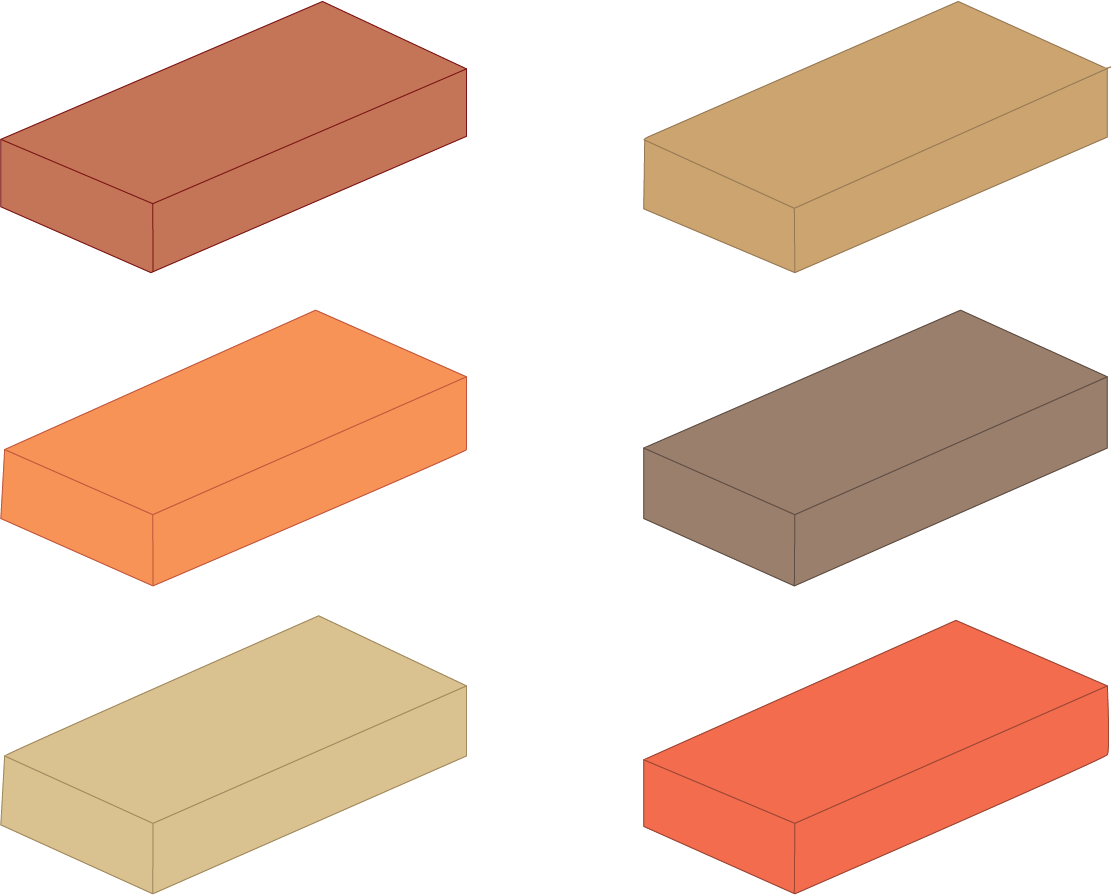Shape of Brick

Solved Example 1: Can you name any two objects which are cuboid in shape?

Ans: Pencil box, Mobile phones are in the shape of cuboids.

Solved Example 2: Is the shape of the refrigerator a cuboid?

Ans: Yes, the refrigerator is in the shape of a cuboid.

### What is a Cuboid?

• It is a three-dimensional shape with three dimensions: length, breadth, and height.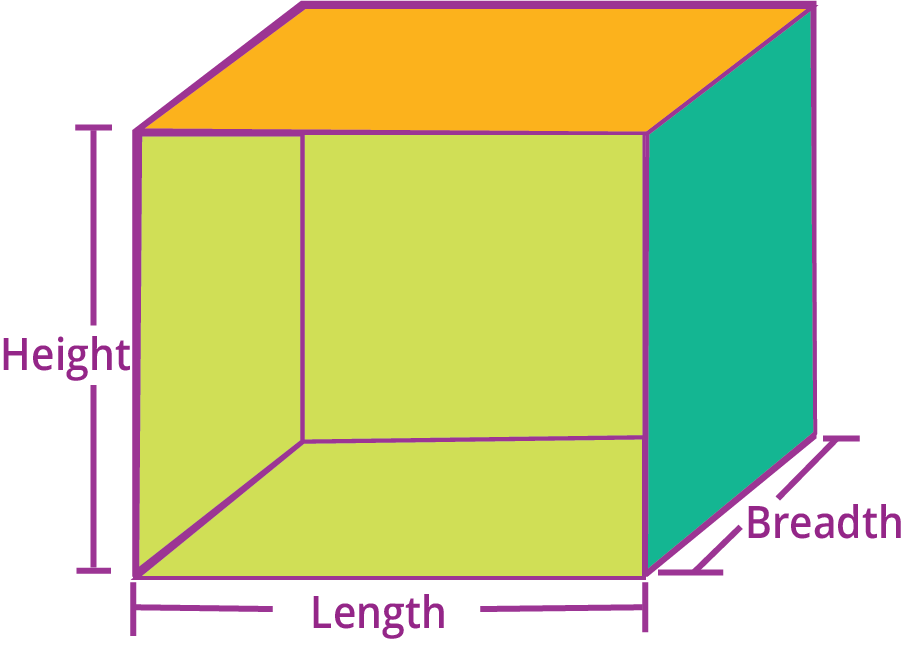Cuboid Dimensions

• It has six faces, eight vertices, and twelve edges.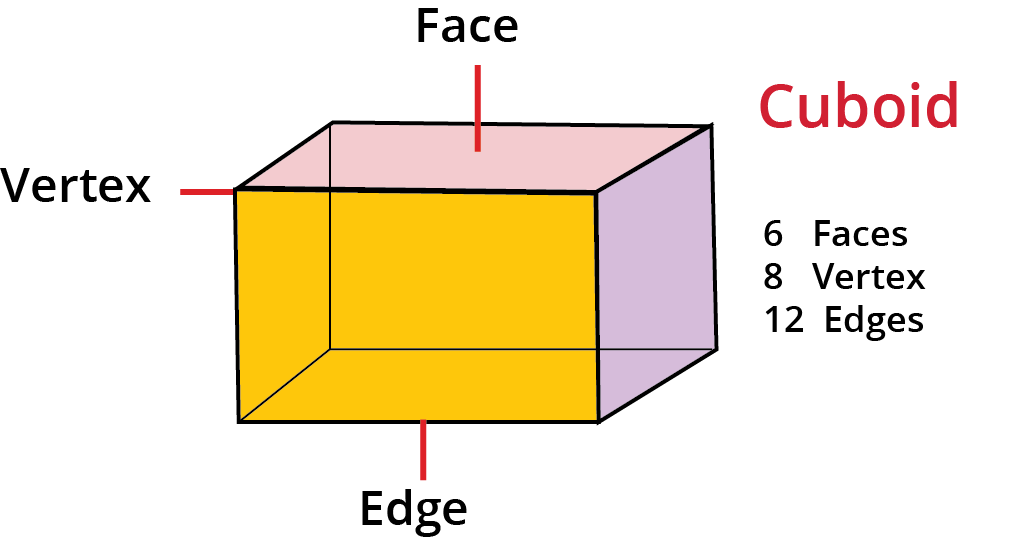Cuboid faces, edges, and vertices

• Real-life examples of cuboids: shoebox, lunch boxes, and mobile phones.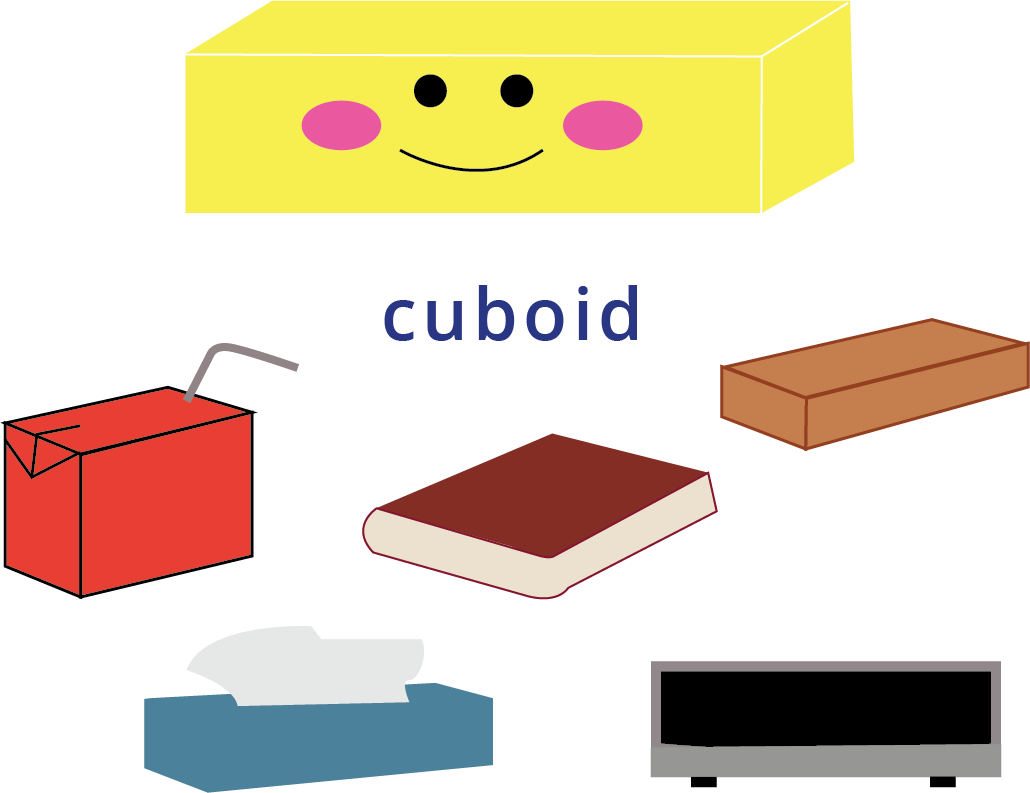Real-life Cuboid examples

### Face of Shape

• A solid's face is the name given to each of its flat surfaces.

• The face of Brick: A brick is in the shape of a cuboid with 6 rectangular faces.

• All the 6 faces of brick are rectangular.

• In the below image, the yellow color indicates 6 rectangular faces of brick.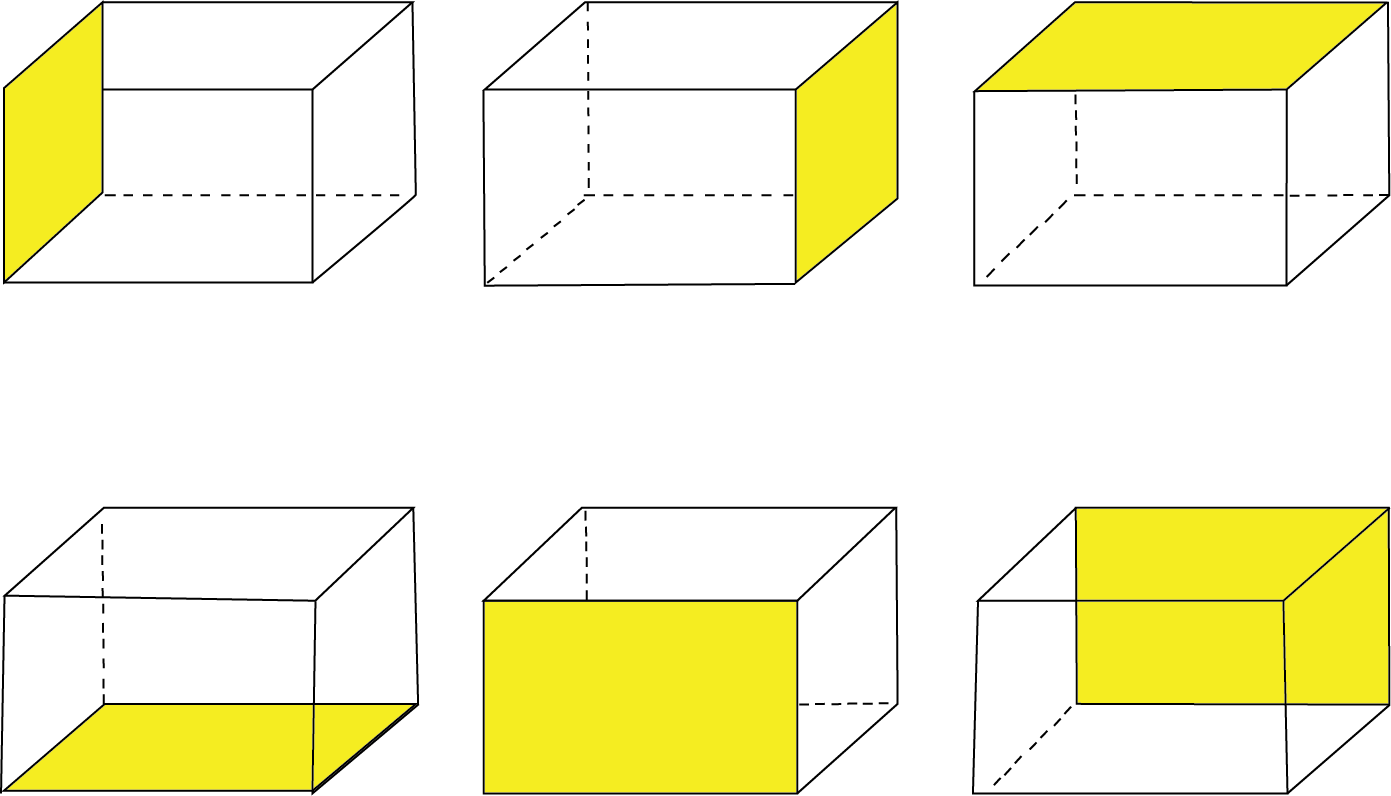Face of brick

Solved Example 1: How many rectangular faces does a matchbox have?

Ans: A matchbox is in the shape of a cuboid, thus having 6 rectangular faces.

Solved Example 2: In the image given below tick the faces of brick?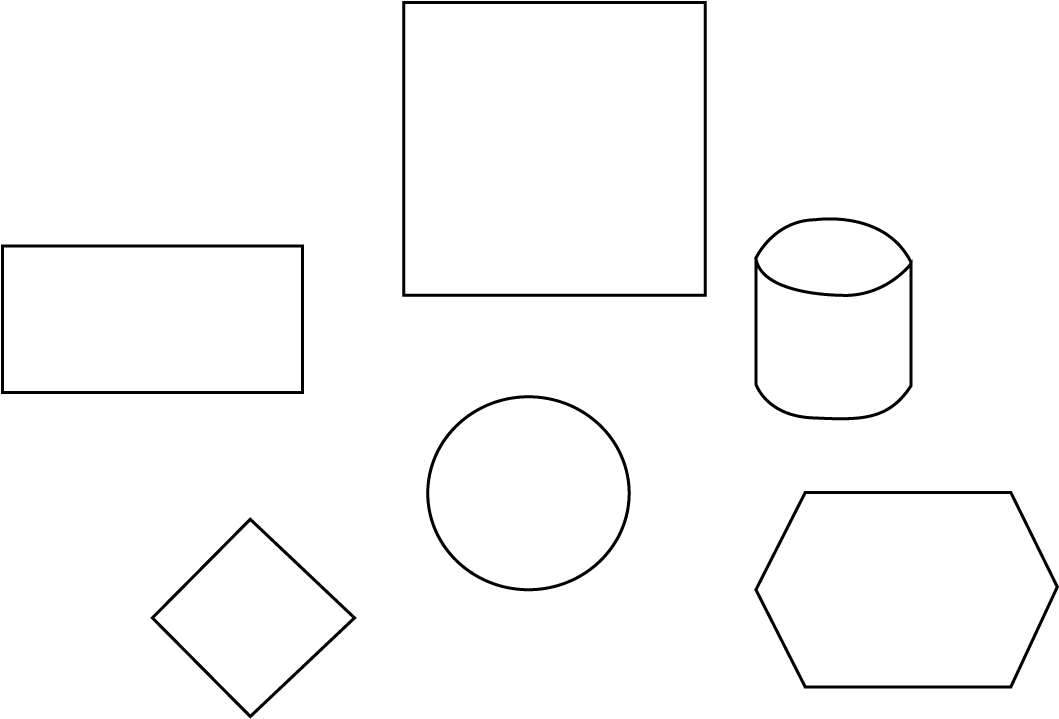Faces of brick

Ans: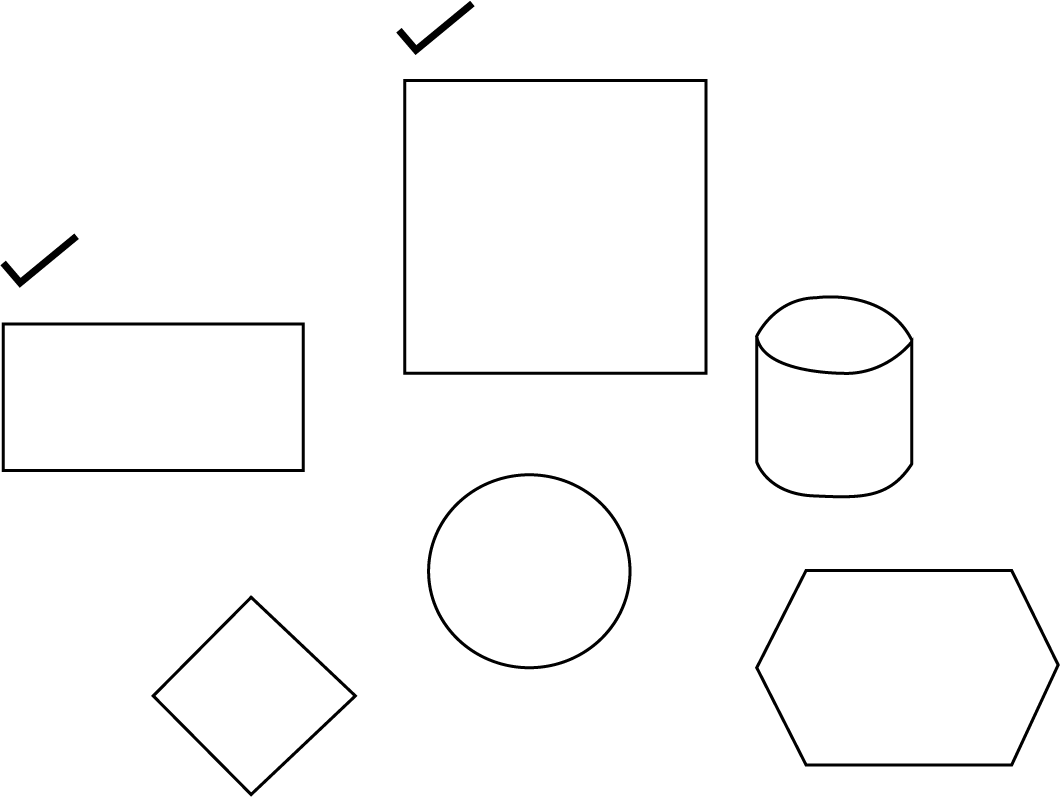Faces of brick

### Symmetry(Mirror Half)

• Any Object (Shape, letter, or number) that can be divided or folded into two halves along a straight line is said to show Symmetry.

•  One half of the symmetrical shape is the mirror image of the other.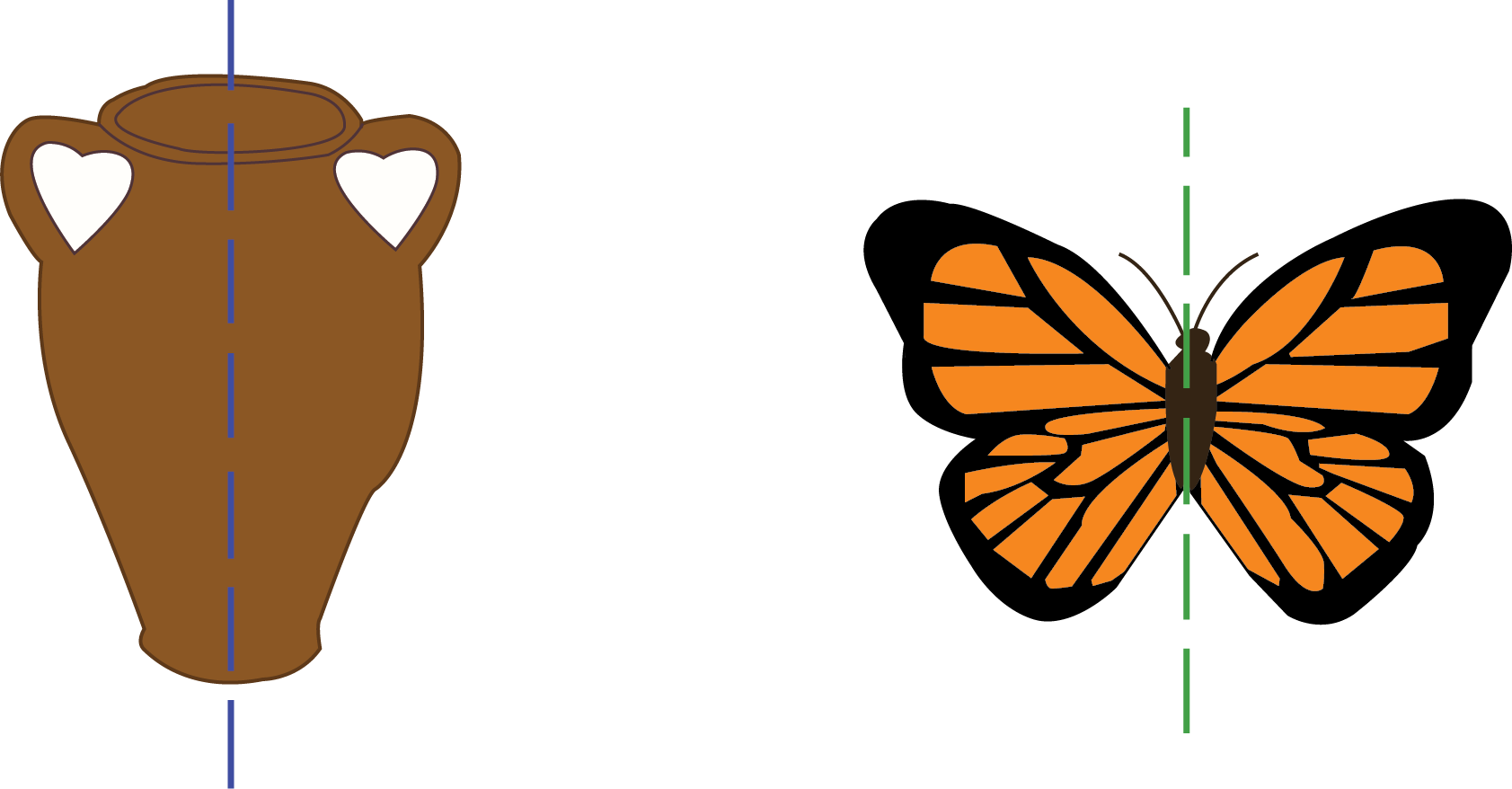Symmetrical objects

Solved Example 1: Can you name any two alphabets which possess symmetry?

Ans: Alphabet (A), and (H) possess symmetry.

Solved Example 2: Can you give any two real objects which possess symmetry?

Ans: Leaf, Lungs in our body possess symmetry.

### Line of Symmetry

• The line that divides a figure into two halves is called the line of symmetry.

• For example, We have a tree here, which we may fold into two equal parts.

• When a figure is folded in half along its symmetry line, the two parts perfectly match.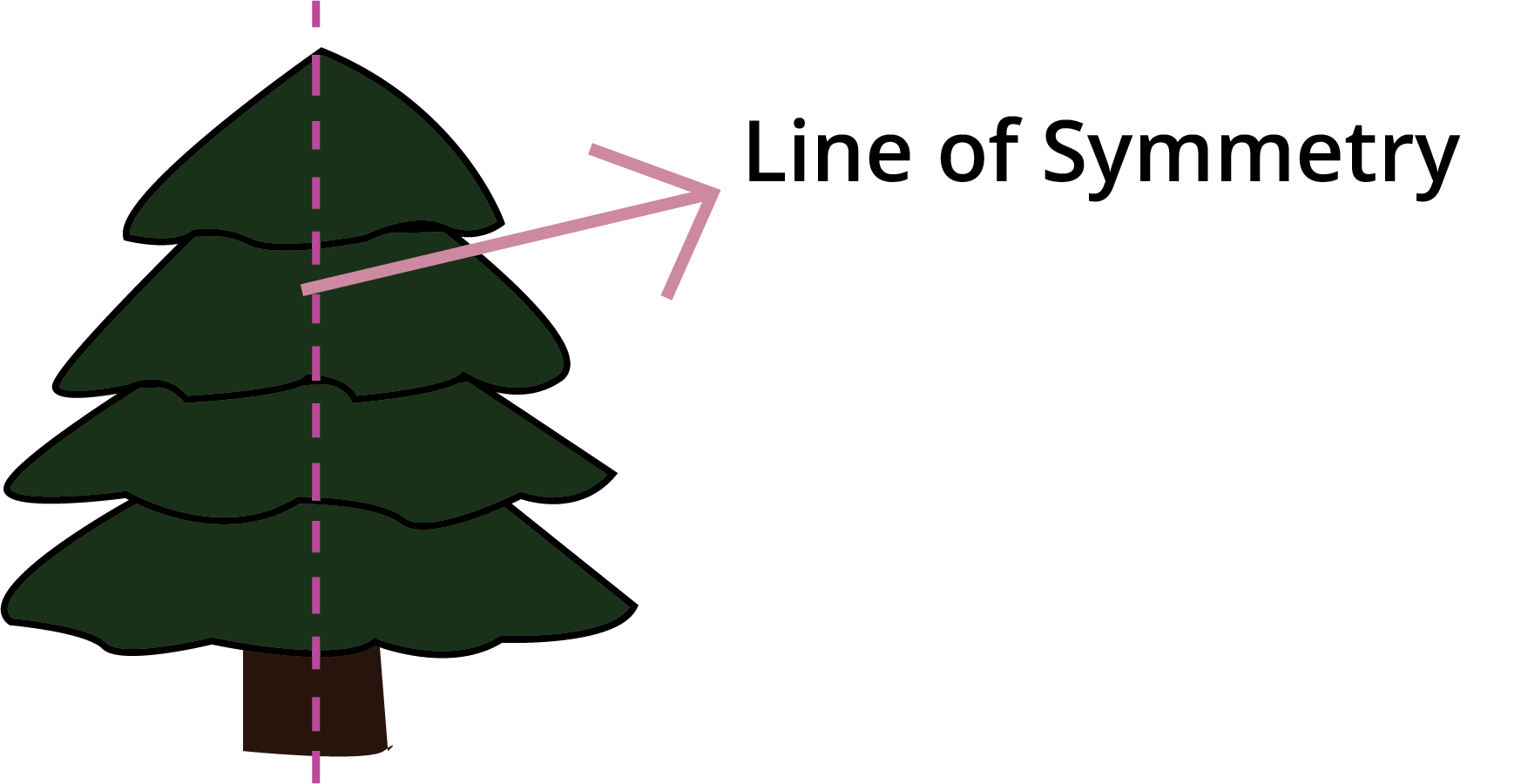Line of symmetry

### Brick Patterns

• By combining different sizes and shapes of bricks, we may create a wide range of patterns and designs.

• Bricks can be used to create wall patterns, tiling patterns, and floor patterns.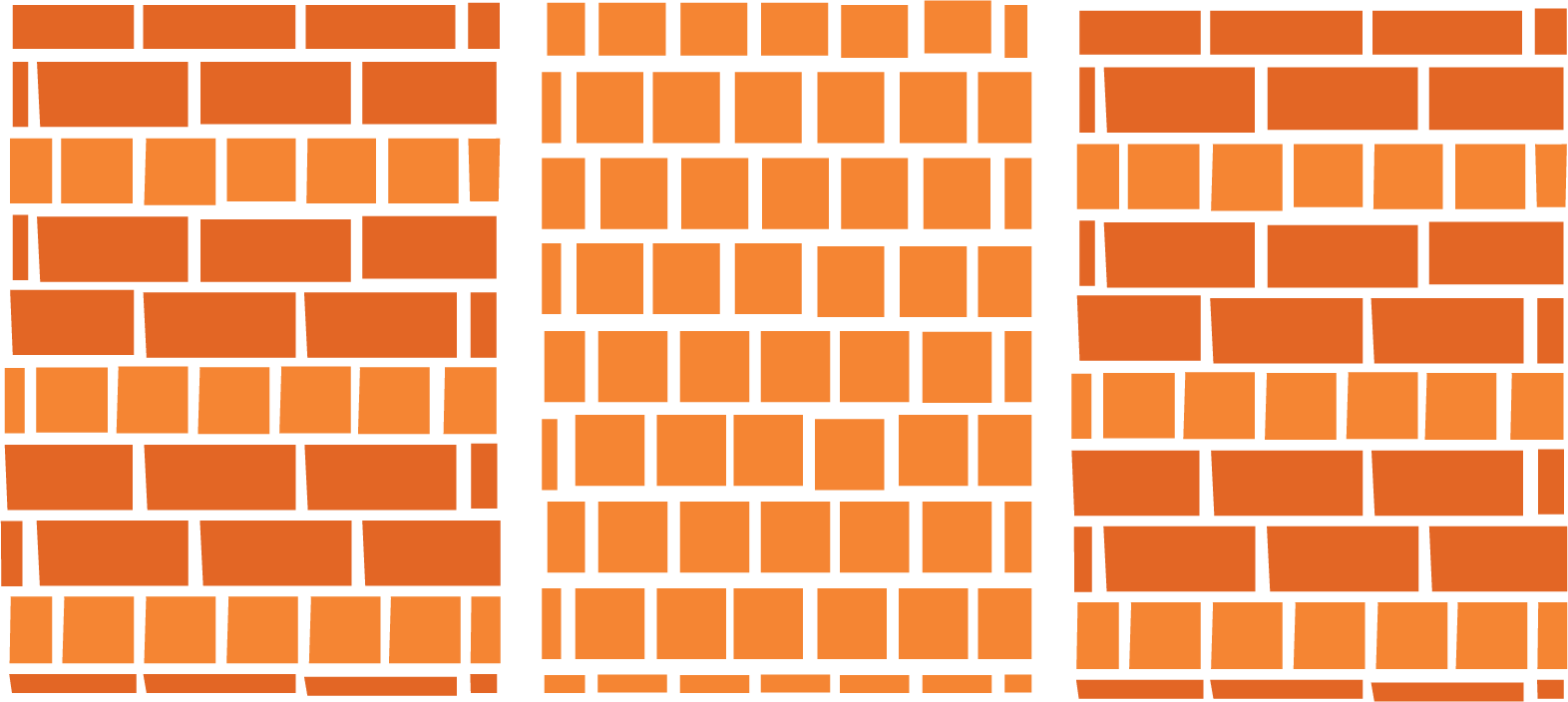Brick patterns

### Different Wall Patterns

• Using bricks we can create different wall patterns.

• Jali patterns and Jharokha patterns are two different types of wall patterns.

• Different shapes and sizes of bricks can be used to create different jali wall patterns and jharokha patterns.

• In the Jali pattern, some equal spaces are created in the wall to create different symmetrical patterns.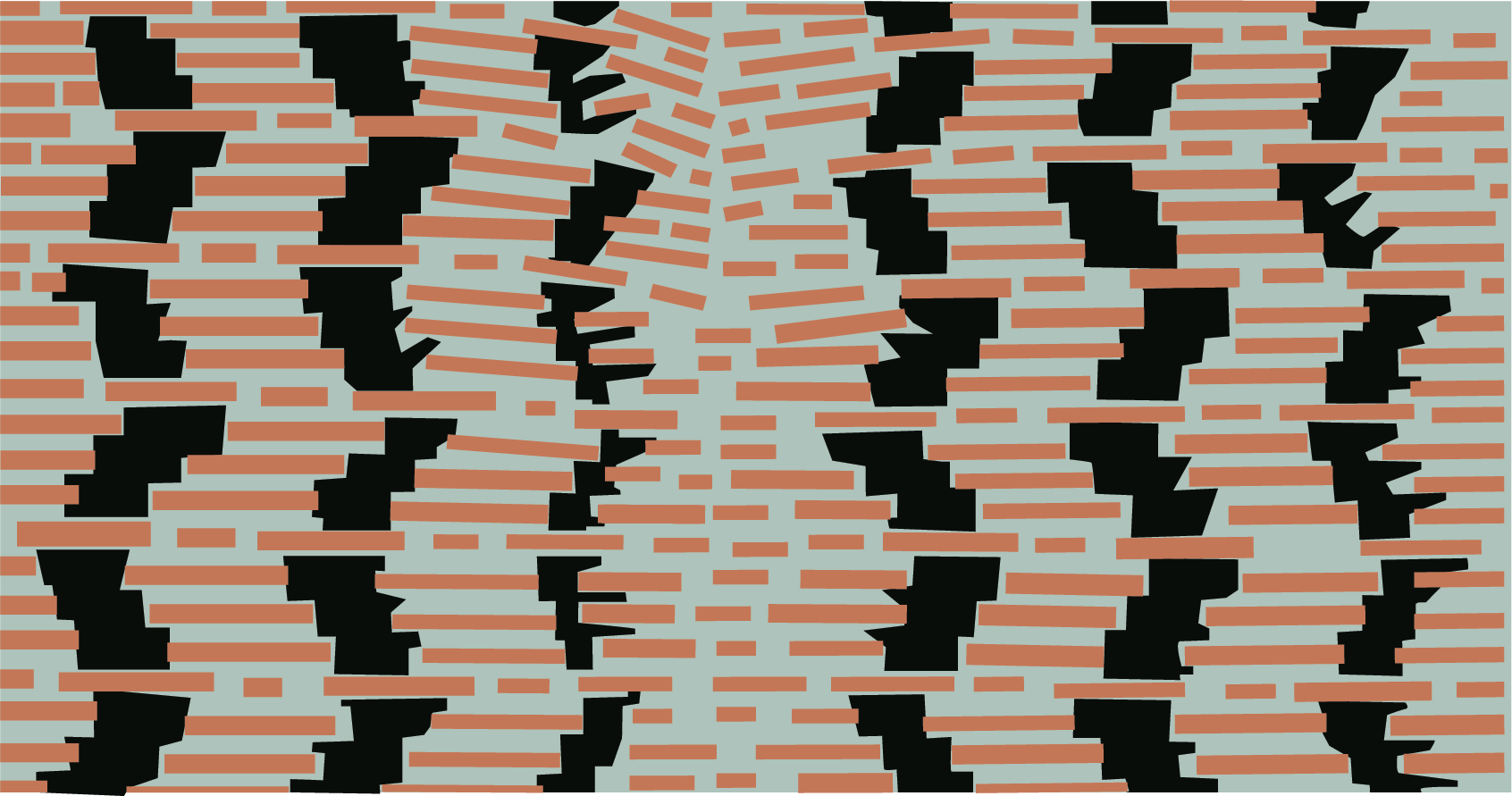Jali Patterns

•  In the Jharokha pattern, space is left in the middle of the wall to create an arch design.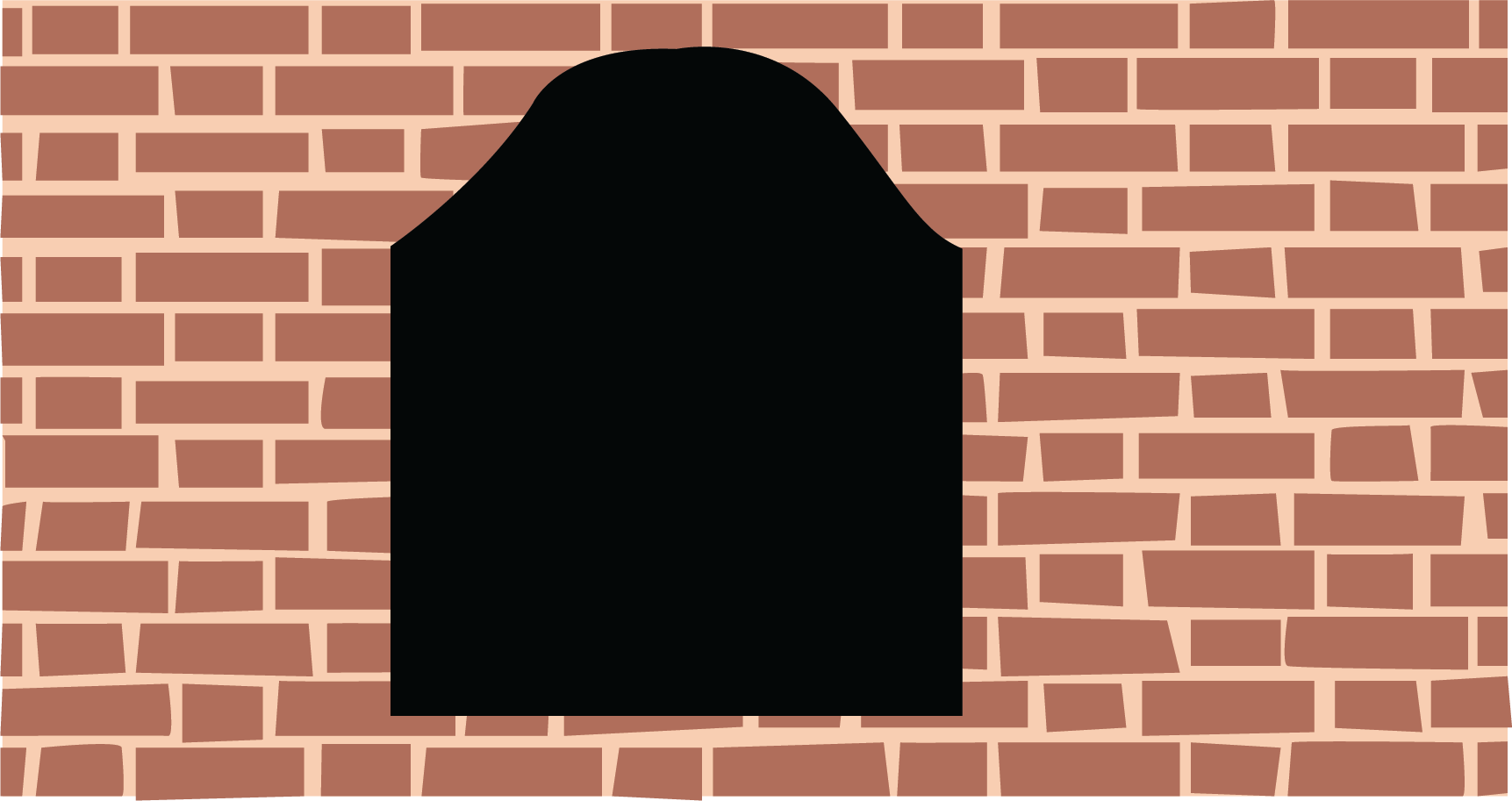Jharokha patterns

### Different Floor Patterns

• Using bricks we can create different floor patterns.

• Circular floor patterns and Symmetrical floor patterns can be created.

• Different shapes and sizes of bricks can be used to create different floor patterns.

• In the circular pattern, bricks are arranged in circular order whereas in the symmetrical pattern bricks are aligned in some symmetrical design.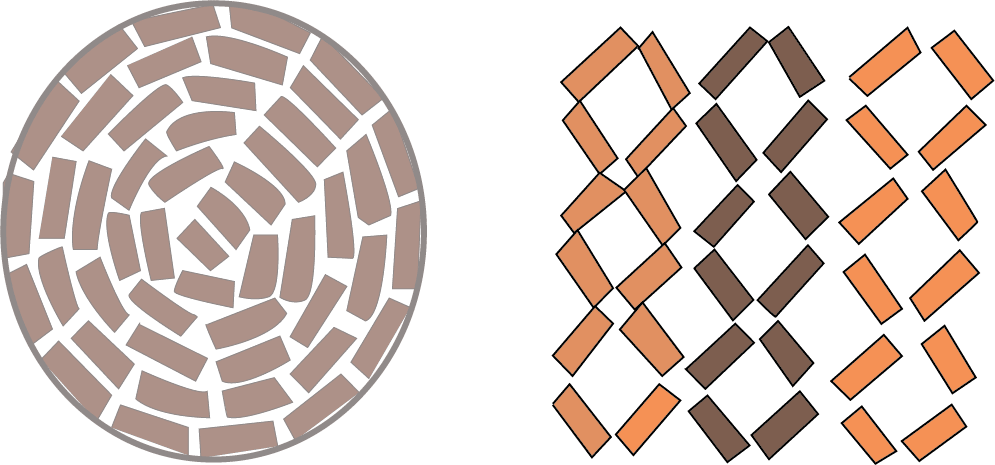Floor patterns

### Arch

• An arch is a curved construction made of bricks or stones with a curved shape.

• For example, Bridge is an arch.Arch

### Cost of Bricks

• To calculate the cost of bricks, we need to multiply the number of bricks purchased by the cost of each brick.

•  Cost of bricks = Number of bricks purchasedcost of each brick.

Solved Example 1: Reena purchased 1000 bricks where each brick costs her ₹12. Find the total cost she paid?

Ans:

• Step1: Write the number of bricks purchased = 1000

• Step2: Write the cost of each brick= ₹12

• Step3: Total cost of bricks = number of bricks purchasedcost of each brick =• So, the Total cost of bricks is ₹1200.

Solved Example 2: Mr. Dixit purchased 5000 bricks where each brick costs her ₹10. Find the total cost she paid?

Ans:

• Step1: Write the number of bricks purchased = 5000

• Step2: Write the cost of each brick= ₹10

• Step3: Total cost of bricks = number of bricks purchasedcost of each brick =• So, the Total cost of bricks is ₹50000.

Solved Example 3: Sam paid ₹1500 per 500 bricks. Find the cost of each brick?

Ans:

• Step1: Write the number of bricks purchased = 500

• Step2: Write the total cost = ₹1500

• Step3: Cost of each brick = Total costTotal number of bricks purchased=• So, the cost of each brick is ₹3.

### Practice Questions:

Q1. From the image given below, answer the following questions: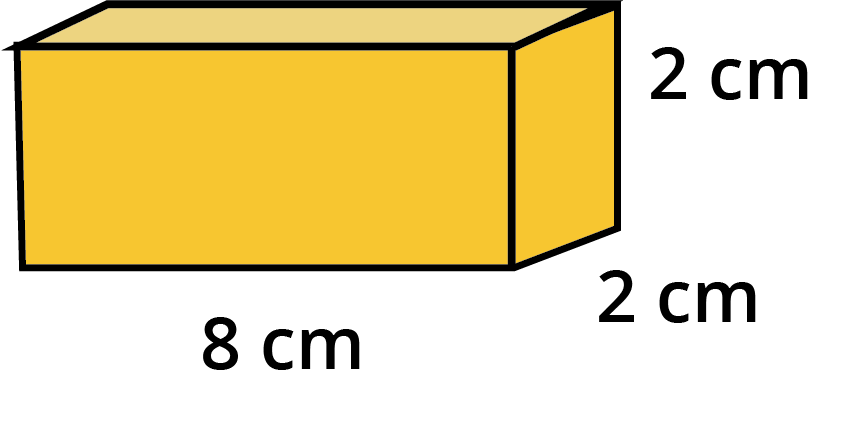Cuboid

• How long is it?

• How wide is it?

• How high is it?

Q2. Make a drawing of the given cuboidal box to show 3 of its faces.Cuboidal box

Q3. Draw the line of symmetry for the following figure: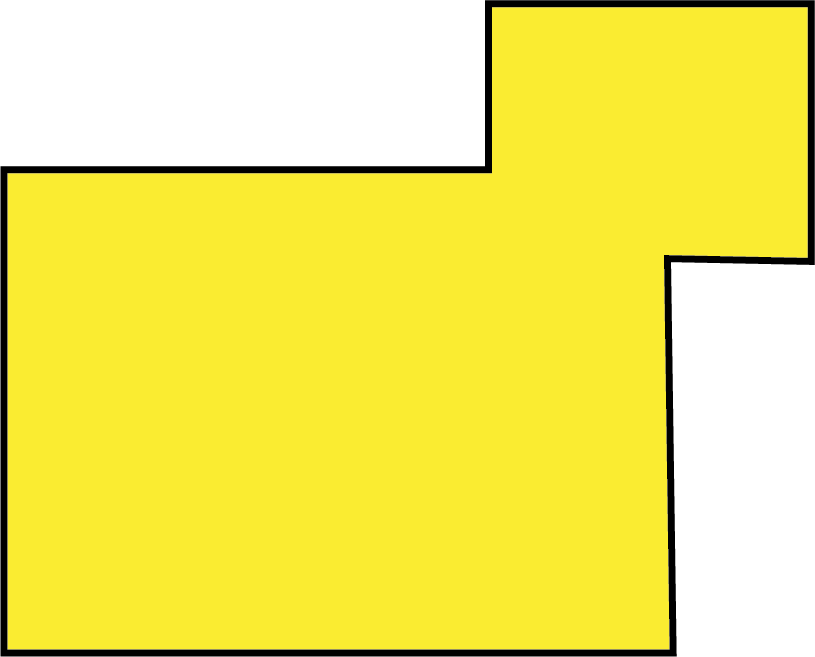Draw the Line of symmetry

Ans 1.

• 8 cm

• 2 cm

• 2 cm

Ans 2.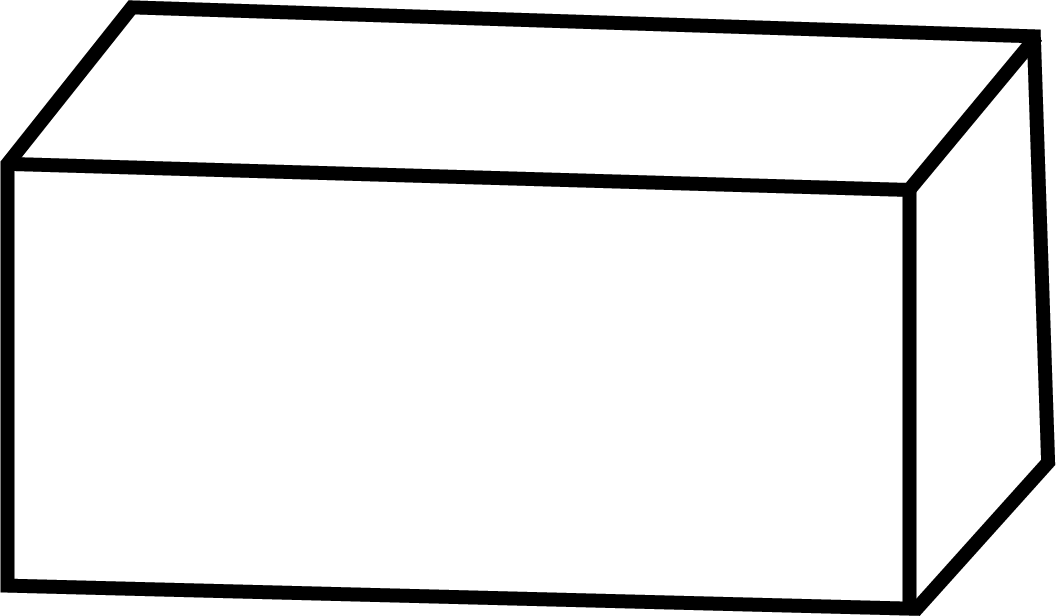Drawing of Cuboidal box

Ans 3.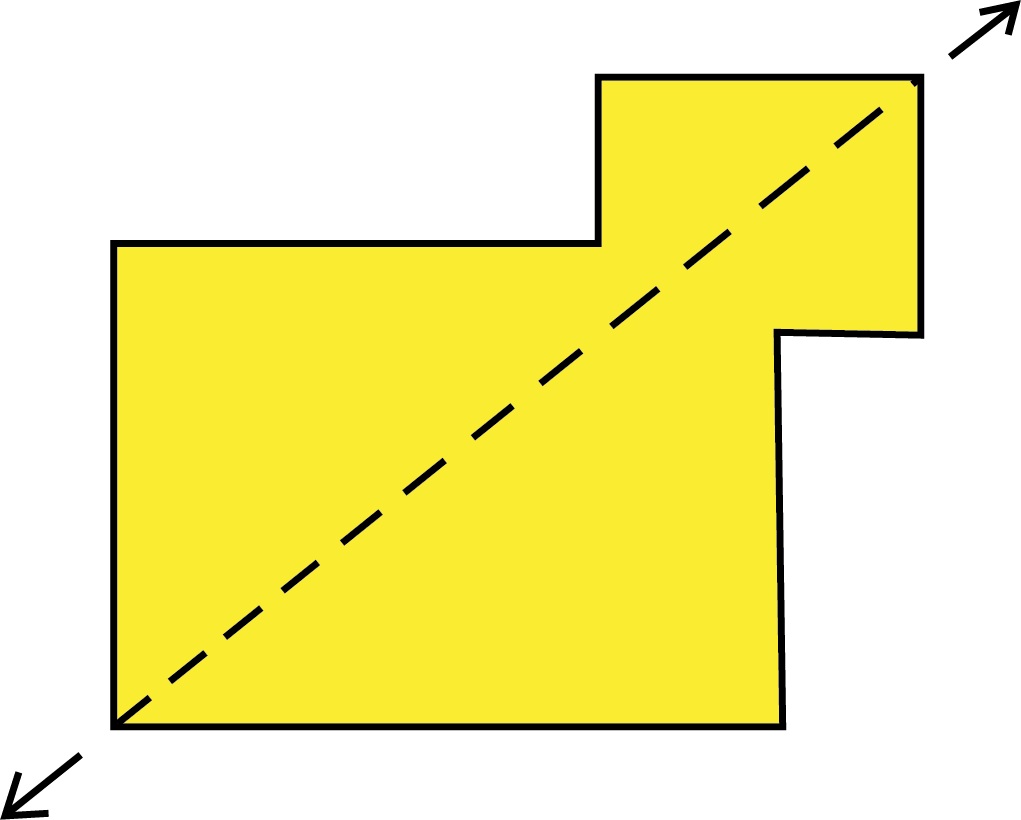Dotted line is the line of symmetry

## Importance of CBSE Class 4 Maths Chapter 1 Building with Bricks

This chapter creates a foundation for understanding 3D objects by using a brick’s shape. A brick is the most common object we see. Using it as a teaching object is a fascinating idea. Here is why this chapter is so important for Class 4 students.

• The explanation of the three different surfaces of a brick enables the students to imagine 3D objects. They have previously learned rectangles and squares. In this chapter, the concepts of 2D shapes will be used to explain a brick.

• Different patterns of bricks are also explained in this chapter along with the shape of a brick. Students will also figure out the stability of brick designs and walls with the help of teachers.

• Children will also learn how to draw a brick from scratch and realise the different types of surface areas or faces of this type of object.

## Benefits of Including Vedantu’s Building with Bricks Revision Notes and Worksheets

The following is a list of benefits that students of Class 4 will gain from referring to our revision notes.

• The shape of brick has been explained in the simplest way. Students will learn the different faces of brick and will understand their utilizations.

• They will also learn how to draw a brick by following the instructions given by the experts.

• They will become experts in recognising the shape of brick from different angles. The explanation of Class 4 Maths Chapter 1 will help them understand the different patterns made using only one type of brick.

• The activities and questions in the exercise are explained by the experts. Students can understand the question formats and understand how to answer them easily.

• Using the revision notes while studying will help students to prepare for the exams faster and better.

• Solving Building with Bricks Class 4 worksheets with answers will also help them practice recalling the concepts and sharpen their answering skills.

## Understanding the Shape of a Brick Becomes Easier

The Building with Bricks summary in the revision notes will help you study the chapter faster before an exam. Understand and memorise the concepts easily by following the explanation given by the experts of Vedantu.

## Conclusion

The Building With Bricks Class 4 Revision Notes CBSE Maths Chapter 1 provide a comprehensive and detailed overview of the chapter, introducing students to the concept of 3D objects and the most primitive and common object, a brick. The notes cover important topics such as the six faces of a brick, different brick patterns, and the different ways bricks are used in modern times. The notes also offer practice exercises and questions that help students test their understanding of the chapter and prepare for their exams. These revision notes are an excellent resource for students who want to improve their math skills and score well in their exams. They are designed by experts according to the CBSE syllabus for Class 4 students and cover all the important topics in the chapter. Overall, the Building With Bricks Class 4 Revision Notes CBSE Maths Chapter 1 are an essential resource for students who want to excel in their mathematics studies.

## FAQs on Building With Bricks Class 4 Notes CBSE Maths Chapter 1 (Free PDF Download)

1. How does solving the Class 4 Maths Chapter 1 worksheet with answers help me?

By solving the questions given in the worksheets compiled by the experts, you will find recalling the studied concepts easier. You will also understand the approaches followed by the experts to answer these questions. Practice solving these worksheets to score better in exams.

2. How many faces does a brick have?

A brick has 6 faces. There are three identical pairs of faces on a brick. Follow the shape of a brick explained in the revision notes to understand this concept better.

3. What is the use of learning brick patterns?

Learning different types of brick patterns will help your mind to imagine new designs and shapes. Imagine using a single type of object to create so many shapes. You will get an answer.